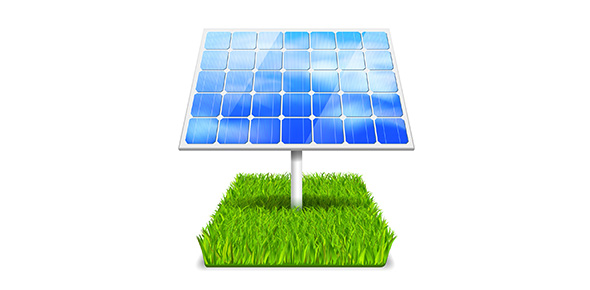# Ch 5 Work & Energy

11 Questions | Total Attempts: 76SettingsRelated Topics
• 1.
Which of the following energy forms is associated with an object in motion? a. potential energy c. nonmechanical energy b. elastic potential energy d. kinetic energy
• A.

A

• B.

B

• C.

C

• D.

D

• 2.
Which of the following energy forms is associated with an object due to its position? a. potential energy c. total energy b. positional energy d. kinetic energy
• A.

A

• B.

B

• C.

C

• D.

D

• 3.
Which of the following energy forms is not involved in hitting a tennis ball? a. kinetic energy c. gravitational potential energy b. chemical potential energy d. elastic potential energy
• A.

A

• B.

B

• C.

C

• D.

D

• 4.
What is the kinetic energy of a 0.135 kg baseball thrown at 40.0 m/s? a. 54.0 J c. 108 J b. 87.0 J d. 216 J
• A.

A

• B.

B

• C.

C

• D.

D

• 5.
The main difference between kinetic energy and potential energy is that a. kinetic energy involves position, and potential energy involves motion. b. kinetic energy involves motion, and potential energy involves position. c. although both energies involve motion, only kinetic energy involves position. d. although both energies involve position, only potential energy involves motion.
• 6.
Gravitational potential energy is always measured in relation to a. kinetic energy. c. total potential energy. b. mechanical energy. d. a zero level.
• 7.
• 8.
Which of the following are not units of power? a. hp c. W b. J d. J/s
• 9.
How much power is required to lift a 2.0 kg mass at a speed of 2.0 m/s? a. 2.0 J c. 9.8 J b. 4.0 J d. 39 J
• 10.
• 11.
A more powerful motor can do a. more work in a longer time interval. b. the same work in a shorter time interval. c. less work in a longer time interval. d. the same work in a longer time interval.